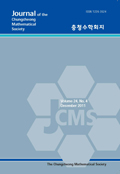상세검색
최근 검색어 전체 삭제
다국어입력
즐겨찾기0KCI등재 학술저널

# MINIMIZATION OF THE DENSE SUBSET

• 등재여부 : KCI등재
• 2020.02
• 33 - 41 (9 pages)
• 2

We introduced the concept of the ε&#8320;-density and the ε&#8320;-dense ace in . This concept is related to the structure of employment. In addition to the double capacity theorem which was introduced in , we need the minimal dense subset. In this paper, we investigate a concept of the minimal ε&#8320;-dense subset in the Euclidean m dimensional space.

1. Introduction

2. Minimization of the dense subset

References